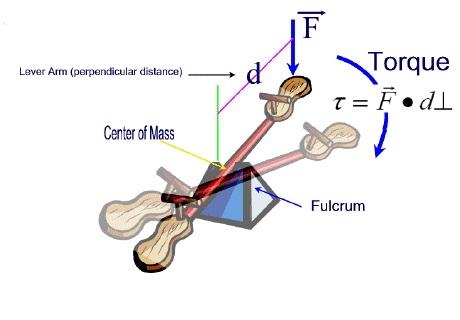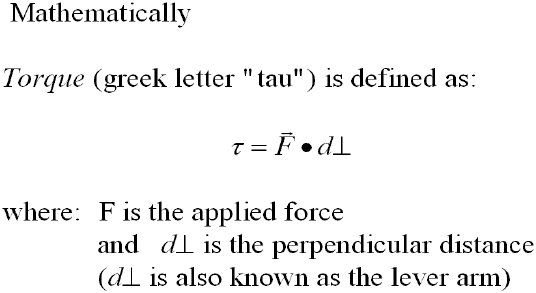### Torque

#### The principle of the lever -- a simple teeter-totterAn object that rotates about a fixed point or about an axis because of a force being applied to it has torque.
Torque is proportional to the applied force and to the perpendicular distance at which this force acts from the given point or axis.• Objects in static equilibrium must satisfy the condition that all forces acting on its center of mass must add up (vectorially) to zero.
• This condition makes sure that all forces that are pulling or pushing the object from one side to the other are balanced.
• There is also another condition that must be met so that the object is in true static equilibrium.
• All torques acting on the center of mass of the object must add up to zero.
• This ensures that the object does not undergo any rotation.
##### In summary:

Conditions for static equilibrium:

1. The sum of all translational forces = zero
2. The sum of all rotational forces = zero

Solutions to Static Equilibrium Problems

1.Solution:

From the Free Body Diagram: